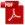### 15.2.11. Inter-Rivet Buckling

Reference: Abbott, Richard. Analysis and Design of Composite and Metallic Flight Vehicle Structures 3 Edition, 2019.

The common method for inter river buckling is based on Euler column behavior and does not include a correction for Poisson’s ratio effect. There is a method defined in  (NACA-TN-3785, 1957) that takes account of the Poisson’s ratio effect and generates allowables about 10% greater than alternative methods.

The ruling equation is an adaptation of the classic plate buckling equation:

The value of the end fixity coefficient is given by the following table:

The plasticity correction factor can be assumed to the same as it is for a panel in compression as defined in section 15.2.4.4.

The cladding reduction factor is defined in section 15.2.9.

A spreadsheet of this method, including plasticity reduction factors, is available here:

### 15.2.11. Inter-Rivet Buckling

Reference: Abbott, Richard. Analysis and Design of Composite and Metallic Flight Vehicle Structures 3 Edition, 2019.

The common method for inter river buckling is based on Euler column behavior and does not include a correction for Poisson’s ratio effect. There is a method defined in  (NACA-TN-3785, 1957) that takes account of the Poisson’s ratio effect and generates allowables about 10% greater than alternative methods.

The ruling equation is an adaptation of the classic plate buckling equation:

The value of the end fixity coefficient is given by the following table: